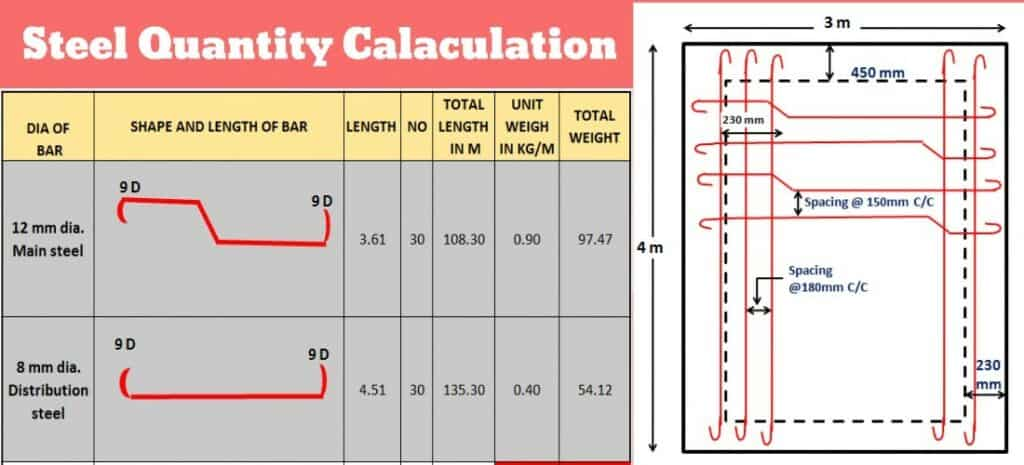How to Calculate Steel Quantity from Drawing

How to Calculate Steel Quantity from Drawing

Estimation of steel quantity is an essential skill of any civil engineer. Every civil engineer must know the method of calculation steel quantity from drawing.

Data requires for estimation of steel quantity:

1) Plan of Section or Element. (Slab, Beam, Column & Foundation)

2) Section and Elevation Details.

3) All dimensions must be clear and co-related.

Steel Quantity Calculation for Slab:

Let, we take one example of slab for steel estimation.

Following Quantity we have to calculate from drawing.

• Cement Concrete for Slab.
• Centering and Shuttering for Slab.
• Weight of 12mm dia. Bars.
• Weight of 8mm dia. Bars.
• Prepare Bar Bending Schedule.

(Note:  Weight of Steel bars 12mm – @ 0.9 kg / m

8 mm – @ 0.4 kg/m,

How to Calculate Steel Quantity from Drawing

Estimation Sheet:

 Sr. No. Item Description No. Length (m) Width/ Breadth (m) Height/ Wt (m) Quantity 1 Cement Concrete for Slab (1:1.5:3): L = 4 + 0.23 + 0.23 = 4.46 m 1 4.46 3.46 0.12 1.85 cu m B = 3 + 0.23 + 0.23 = 3.46

(See drawing in to in dimension (without wall) of slab is given 3 m x 4 m. For calculating Quantity of slab concrete we have to calculate out to out dimension of slab.)

 2 Centering & Shuttering for Slab: Bottom 1 4.00 3.00 – 12.00 cu m Sides 2 4.46 – 0.15 1.34 cu m ( Take 30mm Extra Above Slab Thickness) 2 3.46 – 0.15 1.04 cu m Total 14.38 cu m

(Formwork Quantity is calculated in square meter. There is one bottom portion and 4 side shuttering. For bottom we have in to in dimension of slab 3m x 4m. For Side formwork there is 2 different dimensions, 2 with 4 m side and 2 with 3 m side. For total length we have to add wall width of both sides to get total length.)

Read More: ESTIMATION OF A BUILDING WITH PLAN

How to Calculate Steel Quantity from Drawing

 3 12 mm dia main Steel bars @ 150 mm c/c Alternate bent up. L = 3 + 0.23 + 0.23 + (2 x 9 x 0.012) – (2 x 0.05) L = 3.58 (Straight Length) (For finding out nos. of bar we have to calculate straight length of main bar without adding extra length due to bent up) Span = 4 + 0.23 + 0.23 – (2 x 0.05)          = 4.36 m No. of Bars = (4.36/0.15) + 1                      = 30 nos. Extra Length of Bent up: